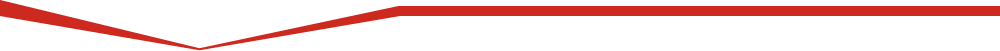Education Help with Education Education Resources UNAVCO Educator Packet Field Geodesy Learning Modules & Activities Majors-level undergraduate Introductory-level undergraduate Secondary- Grades 6-12 Data for Educators Tutorials & Handouts GPS Tutorials 3D Imaging Tutorials Geodesy Education Handouts & KMZs Lab Book Links
Tutorial: Answer

### Answer:Known values
G 6.67 x 10^-11 m^3kg^-1s^-2
m1 5.97 x 10^24 kg
m2 0.001 kg
r 6360001
Unknowns
F ?
a1 ?
a2 ?

## To calculate the force of gravitation between the Earth and the pencil we use the equation for F.

F=Gm1m2/r^2

F=((6.67 * 10^-11)*(5.97 * 10^24)*(0.001))/6360001^2

F=0.009844325 kgm/s^2

## To calculate the acceleration of the Earth towards the pencil we use the equation for a1.

a1=Gm2/r^2

a1=(6.67 * 10^-11)*(0.001)/6360001^2

a1=1.65 * 10^-27 m/s^2

## To calculate the acceleration of the pencil towards the Earth we use the equation for a2.

a2=Gm1/r^2

a2=(6.67 * 10^-11)*(5.97 * 10^24)/6360001^2

a2=9.84 m/s^2

Notice how tiny the acceleration of the Earth is compared to the acceleration of the pencil. This is why we can't tell that the Earth is moving towards the pencil. Also notice how close the acceleration of the pencil is to the accepted average value for the acceleration of gravity on the Earth's surface (9.81 m/s^2)!

Back to Geoid and GPS Receivers

Last modified: Monday, 30-Apr-2018 23:42:13 UTC